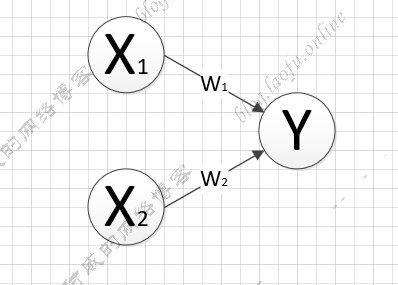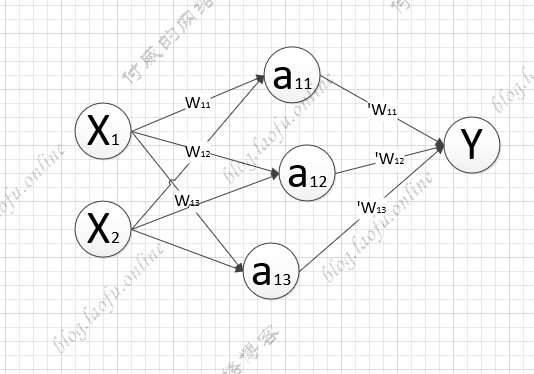# tensorflow的学习笔记--前向传播

### 输入参数w=tf.Variable(tf.random_normal([2,3],stddev=2,mean=0,seed=1))

truncated_normal：去掉过大偏离点的正态分布，如果生成的数据超过了平均值两个标准差，数据将重新生成。

random_uniform：平均分布

tf.zeros:生成全0数组，tf.zeros([3,2],int32) 生成[[0,0],[0,0],[0,0]]
tf.ones:生成全1数组，tf.zeros([3,2],int32) 生成[[1,1],[1,1],[1,1]]
tf.fill:全定值数组，tf.zeros([3,2],6) 生成[[6,6],[6,6],[6,6]]
tf.constant:直接给值，tf.constant([3,2,1]) 生成[3,2,1]

### 神经网络的实现过程

1. 准备数据集，提取特征，作为输入喂给神经网络
2. 搭建NN结构，从输入到输出（先搭建计算图，再用会话执行）
(NN前向传播算法===>>计算输出)
3. 大量特征数据喂给NN,迭代优化NN参数
（NN反向传播算法====>>优化参数训练模型）

4. 使用训练好的模型预测和分类

### 前向传播输入参数X的权重矩阵$W=\left[ \begin{matrix} w_{11} & w_{12} & w_{13} \\ w_{21} & w_{22} & w_{23} \end{matrix} \right]$

隐藏层的矩阵$a=\left[ \begin{matrix} a_{11} & a_{12} & a_{13} \end{matrix} \right] =XW$

隐藏层到输入结果的矩阵：$$’W=\left[ \begin{matrix} w{11} \ w {12} \ w_{13} \ \end{matrix} \right] $$


a=tf.matmaul(X，W)

Y=tf.matmaul(a,'W)

### 分析过程

1. 变量初始化，计算图节点，运算都需要sesion

2. 变量初始化：在session.run函数中，使用tf.global_variables_initializer()

3. 计算图节点运算:在sess.run函数中写入待运算的节点
sess.run(y)

4. 使用tf.placeholder占位，在sess.run函数中用feed_dict喂数据

喂一组数据：

[[3.0904665] [1.2236414] [1.7270732] [2.2305048]]

tensorflow的学习笔记--前向传播

http://blog.laofu.online/2019-03-30-tesnorflow-02/

2019-03-30

2019-03-30